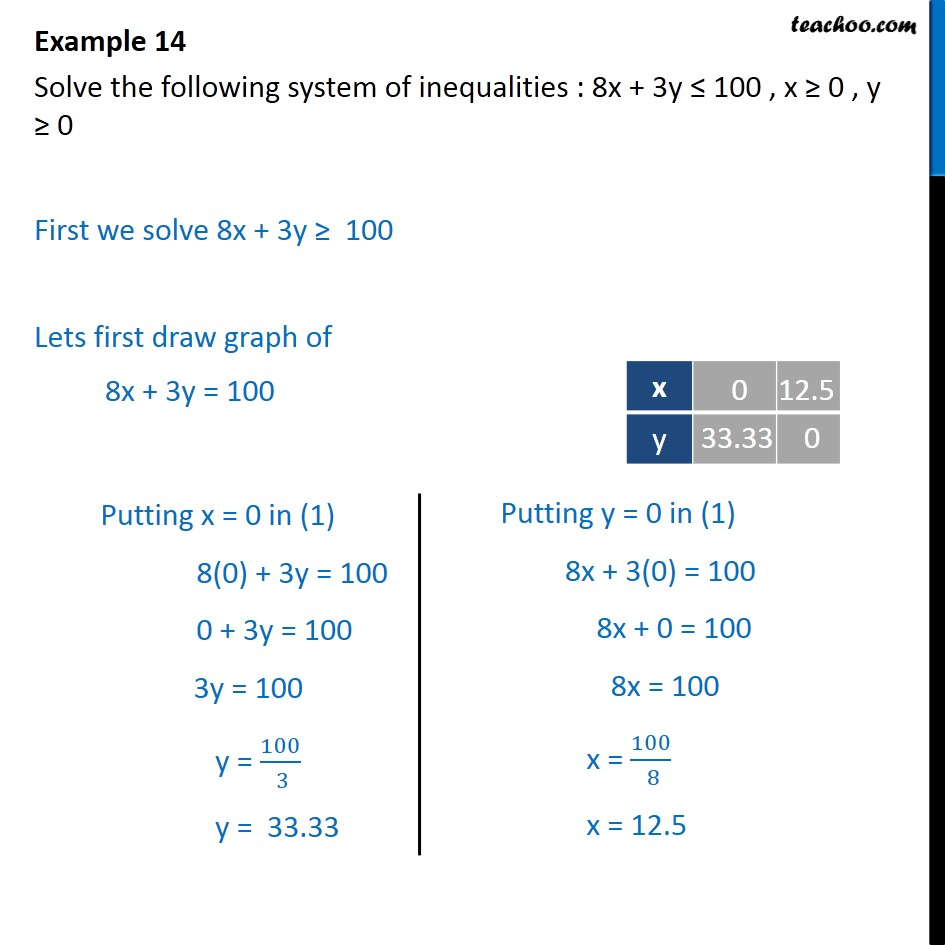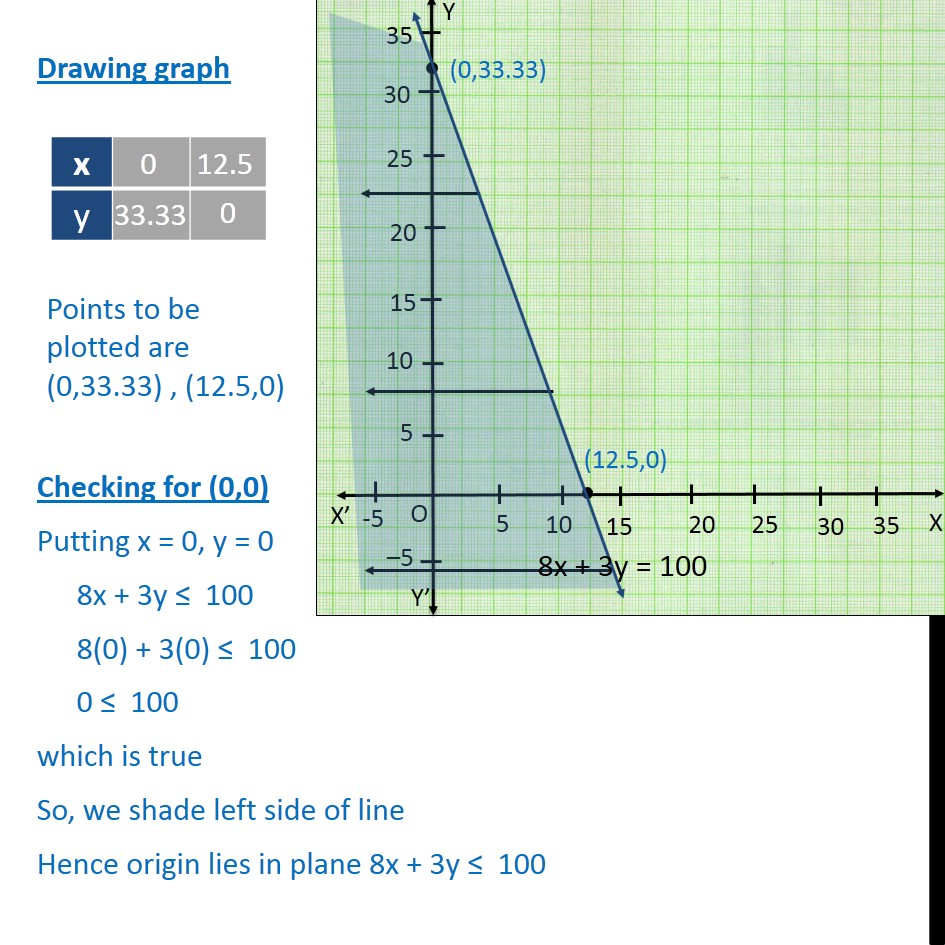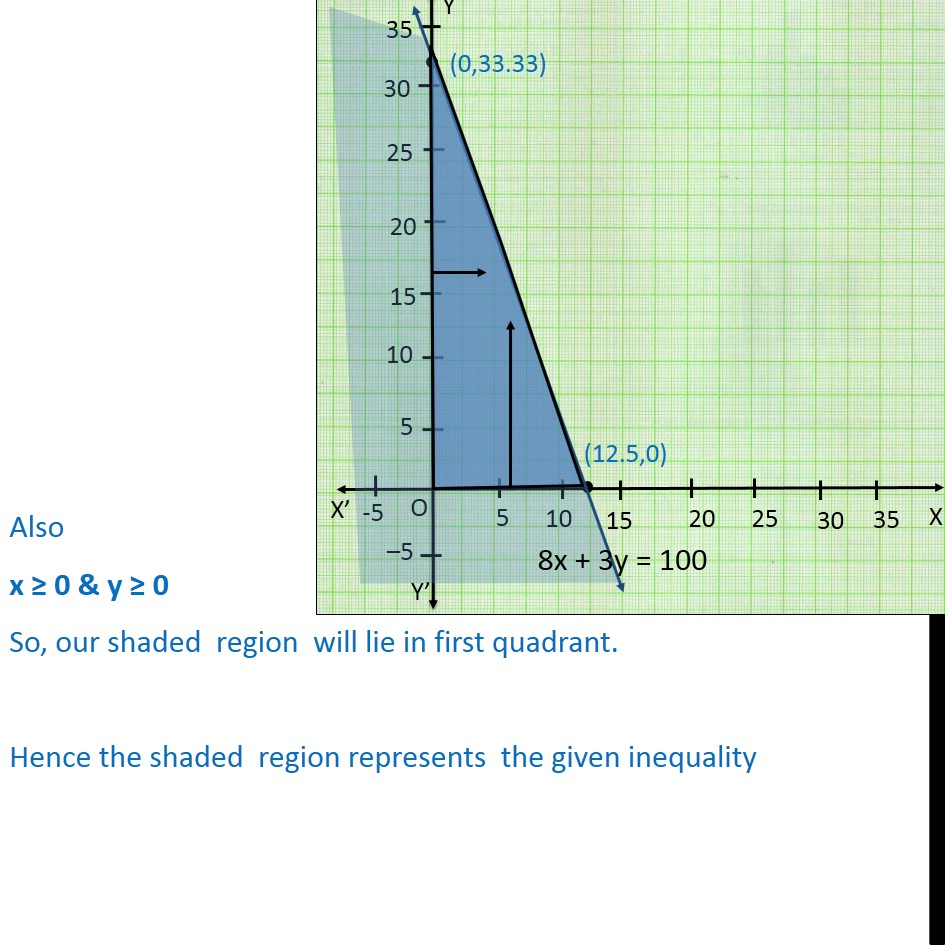1. Chapter 6 Class 11 Linear Inequalities (Term 2)
2. Serial order wise
3. Examples

Transcript

Example 14 Solve the following system of inequalities : 8x + 3y ≤ 100 , x ≥ 0 , y ≥ 0 First we solve 8x + 3y ≥ 100 Lets first draw graph of 8x + 3y = 100 Drawing graph Checking for (0,0) Putting x = 0, y = 0 8x + 3y ≤ 100 8(0) + 3(0) ≤ 100 0 ≤ 100 which is true So, we shade left side of line Hence origin lies in plane 8x + 3y ≤ 100 Also x ≥ 0 & y ≥ 0 So, our shaded region will lie in first quadrant. Hence the shaded region represents the given inequality

Examples

Chapter 6 Class 11 Linear Inequalities (Term 2)
Serial order wise